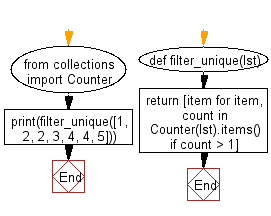﻿ Python: Create a list with the unique values filtered out - w3resource# Python: Create a list with the unique values filtered out

## Python List: Exercise - 224 with Solution

Write a Python program to create a list with the unique values filtered out.

• Use collections.Counter to get the count of each value in the list.
• Use a list comprehension to create a list containing only the non-unique values.

Sample Solution:

Python Code:

``````from collections import Counter
def filter_unique(lst):
return [item for item, count in Counter(lst).items() if count > 1]
print(filter_unique([1, 2, 2, 3, 4, 4, 5]))
```
```

Sample Output:

```[2, 4]
```

Flowchart:## Visualize Python code execution:

The following tool visualize what the computer is doing step-by-step as it executes the said program:

Python Code Editor:

Have another way to solve this solution? Contribute your code (and comments) through Disqus.

What is the difficulty level of this exercise?

Test your Python skills with w3resource's quiz

﻿

## Python: Tips of the Day

Floor Division:

When we speak of division we normally mean (/) float division operator, this will give a precise result in float format with decimals.

For a rounded integer result there is (//) floor division operator in Python. Floor division will only give integer results that are round numbers.

```print(1000 // 300)
print(1000 / 300)```

Output:

```3
3.3333333333333335```